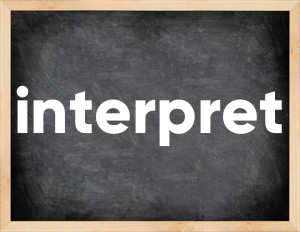# Interpret past tenseThe English verb 'interpret' is pronounced as [ɪnˈtəːprɪt].
Related to: regular verbs.
3 forms of verb interpret: Infinitive (interpret), Past Simple - (interpreted), Past Participle - (interpreted).

## Here are the past tense forms of the verb interpret

👉 Forms of verb interpret in future and past simple and past participle.
❓ What is the past tense of interpret.

## Interpret: Past, Present, and Participle Forms

Base Form Past Simple Past Participle
interpret [ɪnˈtəːprɪt]

interpreted [ɪnˈtɜːprɪtɪd]

interpreted [ɪnˈtɜːprɪtɪd]

## What are the 2nd and 3rd forms of the verb interpret?

🎓 What are the past simple, future simple, present perfect, past perfect, and future perfect forms of the base form (infinitive) 'interpret'?

### Learn the three forms of the English verb 'interpret'

• the first form (V1) is 'interpret' used in present simple and future simple tenses.
• the second form (V2) is 'interpreted' used in past simple tense.
• the third form (V3) is 'interpreted' used in present perfect and past perfect tenses.

## What are the past tense and past participle of interpret?

The past tense and past participle of interpret are: interpret in past simple is interpreted, and past participle is interpreted.

### What is the past tense of interpret?

The past tense of the verb "interpret" is "interpreted", and the past participle is "interpreted".

### Verb Tenses

Past simple — interpret in past simple interpreted (V2).
Future simple — interpret in future simple is interpret (will + V1).
Present Perfect — interpret in present perfect tense is interpreted (have/has + V3).
Past Perfect — interpret in past perfect tense is interpreted (had + V3).

### interpret regular or irregular verb?

👉 Is 'interpret' a regular or irregular verb? The verb 'interpret' is regular verb.

## Examples of Verb interpret in Sentences

•   We decided to interpret it as a gesture of mercy (Past Simple)
•   Courts usually interpreted these provisions arbitrarily and inconsistently. (Past Simple)
•   Be careful, any of your words can be interpreted as an admission of guilt. (Present Simple)
•   I don't understand how should we interpret this law. (Present Simple)
•   How can you interpret Martha's behavior? (Present Simple)
•   I had to interpret the speech for them, because they didn't understand English. (Past Simple)
•   Every actor interprets the same role differently. (Present Simple)
•   And left me completely in the dark as to how to interpret it. (Past Simple)
•   We need someone to interpret to us what these results mean. (Present Simple)
•   Can you interpret what the VIPs are saying? (Present Simple)

Along with interpret, words are popular bank and pair.

Verbs by letter: , , , , , , , , , , , , , , , , , , , , , , , , .